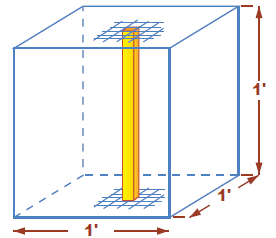# Basic Pressure Definitions

Understanding pressure is very important when dealing with well control issues (Click Here). The pressure can be defined as the force exerted or placed on a unit of area such pounds per square inch (psi).

The fluid exerts a pressure which is related to the density of the fluid and the height of the fluid column. Density is usually measured in pounds per gallon (ppg) or kilograms per cubic meter (kg/m3). The pressure gradient is used to determine the level of pressure at any point. It is defined as the pressure increase per unit of depth. It is normally expressed as the force exerted by the fluid per unit of length. It is measured in pounds per square inch per foot (psi/ft).

The density can be converted to pressure gradient using a conversion factor (0.052) which is derived from the following principle: a one foot cube is filled with 7.48 gallons of fluids which has the density of one pound per gallons, then the total weight of the cube is 7.78 pounds. The weight exerted on an area of one square inch can be found by dividing the total weight of the cube by 144:
7.48÷144=0.051944Fig 01- Conversion Factor Principle

The pressure gradient of any fluid is deduced by multiplying the density of the fluid by 0.052.

## True vertical depth TVD and the measured depth MD

In figure 02, you can notice that both wells A and B have reached the same point if we pass a horizontal plan through the both points A and B at the depth of the 10000 ft, but in fact the drilling bit has not passed the same passage for the well A and B. the bit at the well A has drilled 10000 ft but in the well bit has drilled 11650 ft. we say the well B has a true vertical depth of 10000 ft and a measured depth of 11650 ft. for the well A the true vertical depth and measured depth are the same.Fig 02- TVD vs MD

Hydrostatic pressure is the pressure exerted by a column of fluid at a certain depth at the static situation.
Or it can be calculated by
It has to be mentioned here, that the pressure is calculated using the true vertical depth.

## U-Tubing Effect

It is often helpful to visualize the well as a U-tube. One column of the tube represents the annulus and the other column represents the drill string in the well. The bottom of the U-tube represents the bottom of the well. The fluids create hydrostatic pressures in the both sides. If there is 10 ppg fluid in the pipe and annulus, hydrostatic pressures would be equal and the fluid would be static. However, what will happen if fluid in the annulus is heavier than the fluid in the string? The heavier fluid in the annulus exerts more hydrostatic pressure downward pushing the fluid to flow into the string, displacing some of the lighter fluid out of the string, causing a back flow at surface. The fluid level will fall in the annulus till getting to the pressures equalization. When there is a difference in the hydrostatic pressures, the fluid will try to reach balance point. This is called U-tubing, and it explains why there is often flow from the pipe when making connections. This is often evident when drilling fast because the effective density in the annulus is increased by cuttings.Fig 03- U Tubing Analogy

## Pore (Formation) Pressure

Formation or pore pressure is the pressure within the pore spaces of formation rock. This pressure can be affected by the weight of the overburden (rock layers) above the formation which exerts pressure on both grains and pore fluids. If the formation fluids are free to move, the grains lose some of their support and become closer leading to what it is called compaction.

The normal trend of the formation pressure equals to hydrostatic pressure generated by a vertical column of fresh water from the designed depth up to the surface. The pressure gradient of fresh water varies from 0.433 psi/ft to 0.465 psi/ft.

Abnormal formation pressure can be generated for many reasons such as the presence of faults and salt dome. During the abnormally pressure formations development, the fluids flow can be restricted or stopped which can affect the normal trend of pressure. The pressure gradient of the abnormally pressurized formations exceeds the normal gradient of 0.465 psi/ft. controlling such formations can lead to mud weight increase till the level of 20 ppg. (Click Here)

Also, the formation pressure can be subnormal when the pressure gradient becomes lower than 0.433 psi/ft. it can be developed when the overburden is stripped away or when the formation is depleted by evaporation or capillary action.

## Fracture Pressure

Fracture pressure is the level of pressure it takes to permanently deform (fail or split) the rock structure of a formation. Overcoming formation pressure is usually not sufficient to cause fracturing. If pore fluid is free to move, a slow rate of entry into the formation will not cause fractures. If pore fluid cannot move out of the way, fracturing and permanent deformation of the formation can occur. Fracture pressure can be expressed as a gradient (psi/ft), a fluid density equivalent (ppg), or by calculated total pressure at the formation (psi). Fracture gradients normally increase with depth due to increasing overburden pressure. Deep, highly compacted formations can require very high fracture pressures to overcome the existing formation pressure and resisting rock structure. Loosely compacted formations, such as those found offshore in deep water, can fracture at low gradients. Fracture pressures at any given depth can vary widely because of the geology of the area.

1.1.